# RS Aggarwal Solutions for Class 9 Chapter 18: Mean, Median and Mode of Ungrouped Data Exercise 18A

## RS Aggarwal Solutions for Class 9 Maths Exercise 18A PDF

RS Aggarwal Solutions are created with the aim of strengthening skills and conceptual knowledge among students. Understanding the topics in Class 9 is important as some of the topics are repeated in higher grades as well. Accurate solutions are created by subject experts based on the RS Aggarwal textbook of current CBSE syllabus. The students can use PDF of solutions as reference material from exam point of view. RS Aggarwal Solutions for Class 9 Maths Chapter 18 Mean, Median and Mode of Ungrouped Data Exercise 18A are provided here.

## RS Aggarwal Solutions for Class 9 Chapter 18: Mean, Median and Mode of Ungrouped Data Exercise 18A Download PDF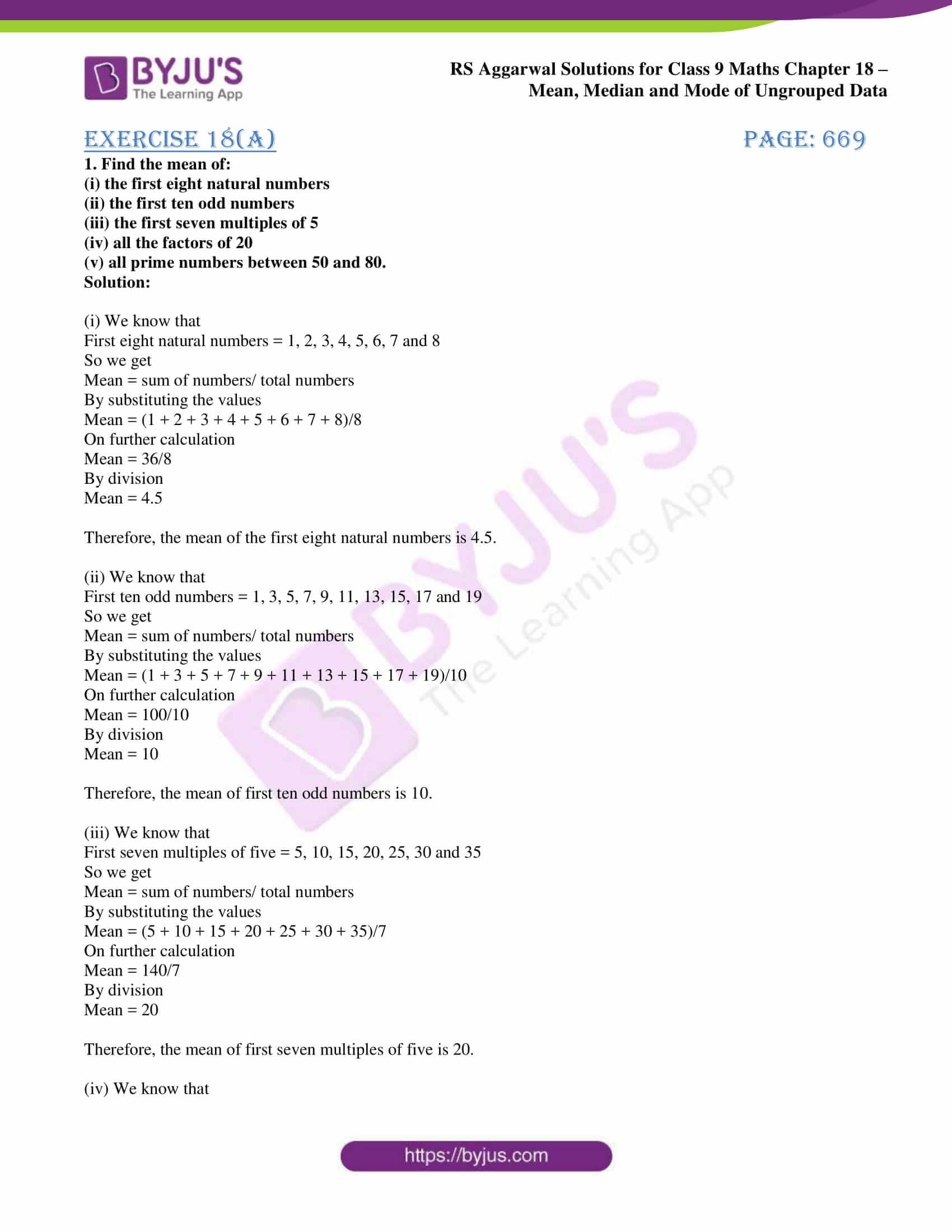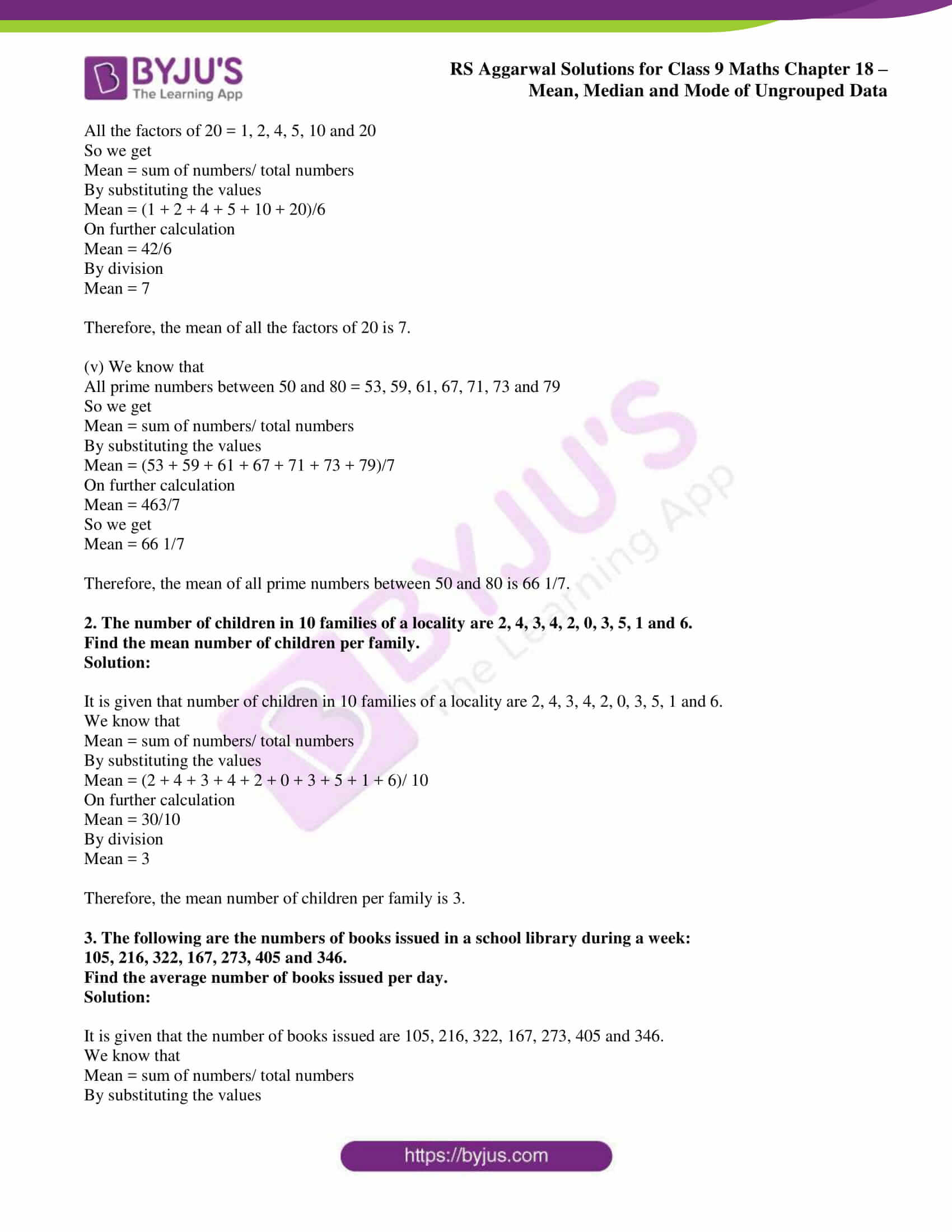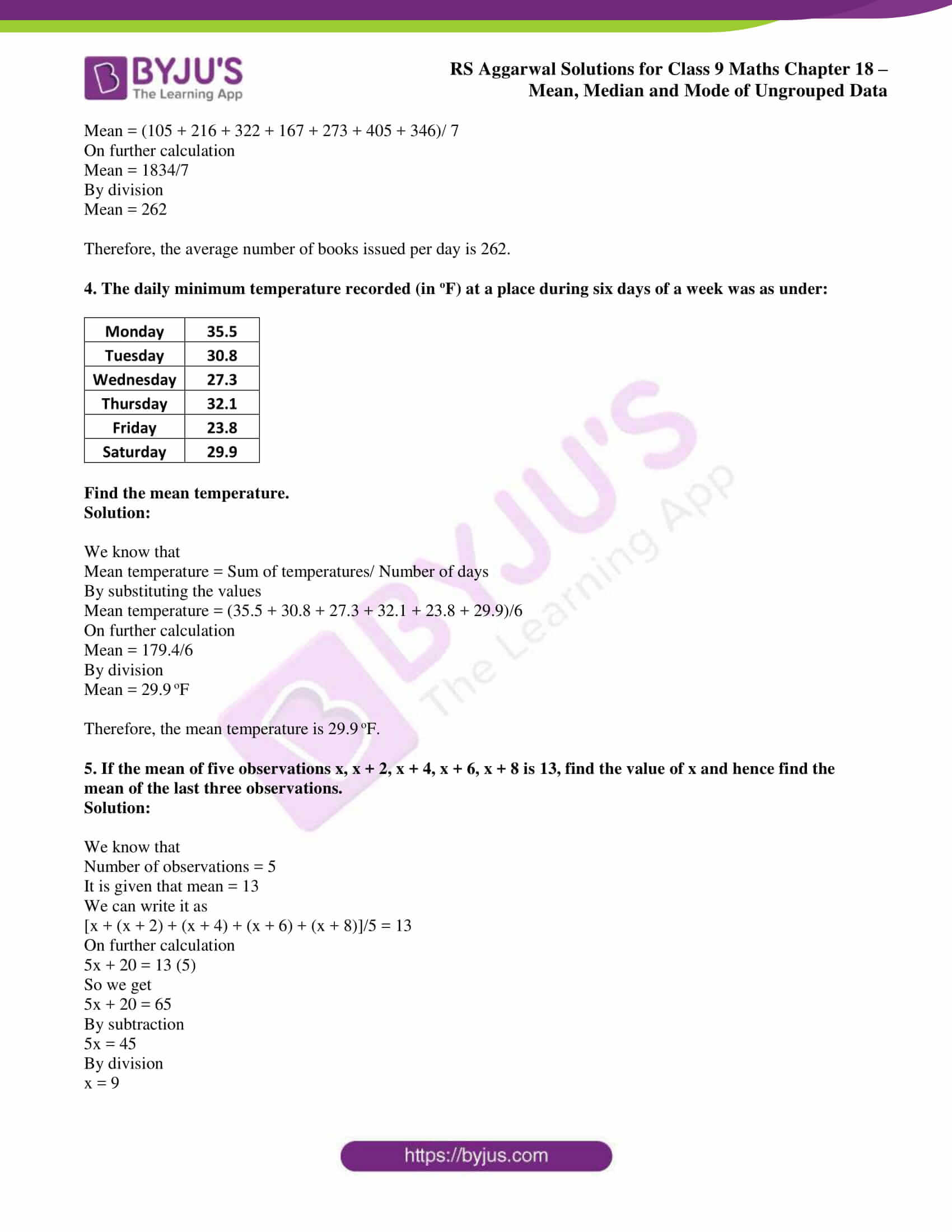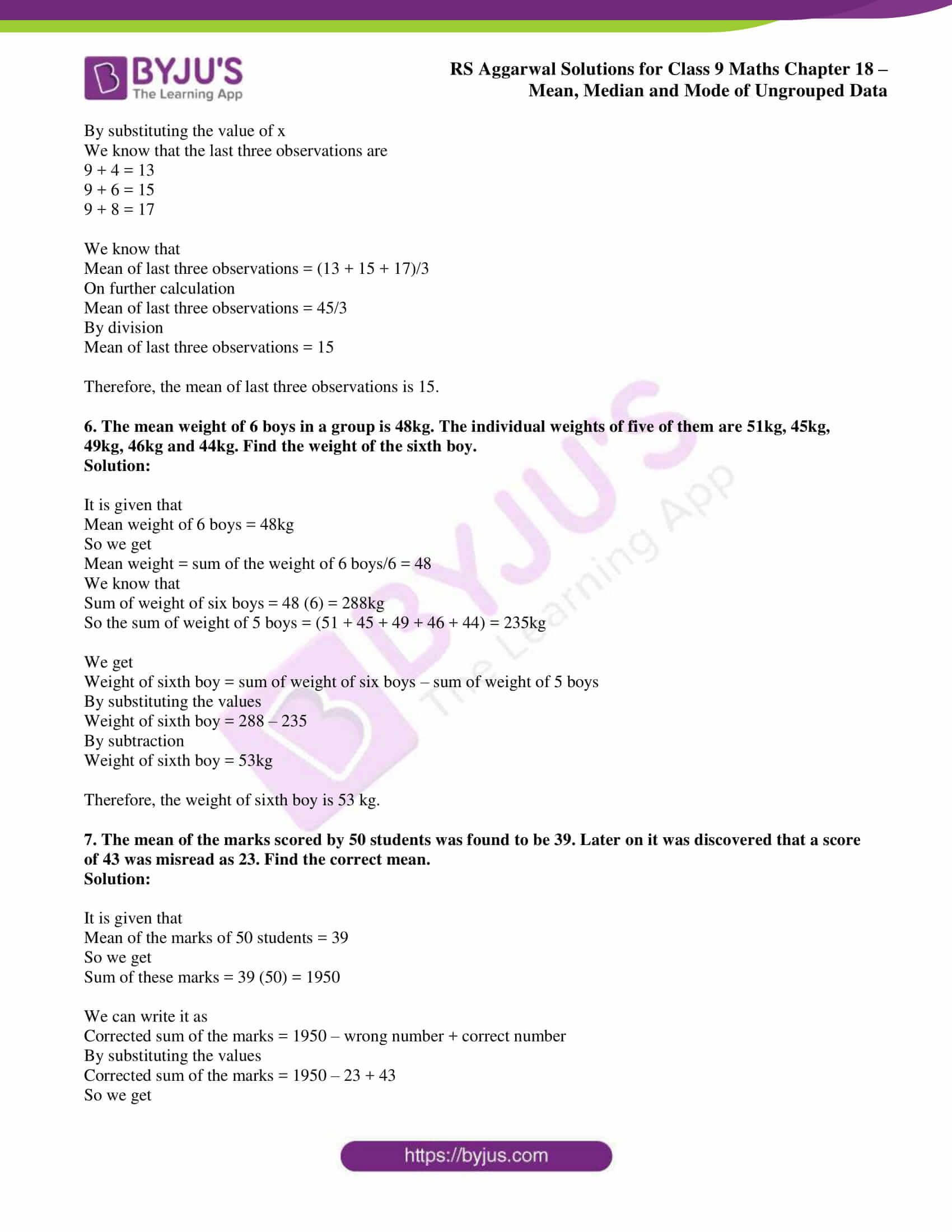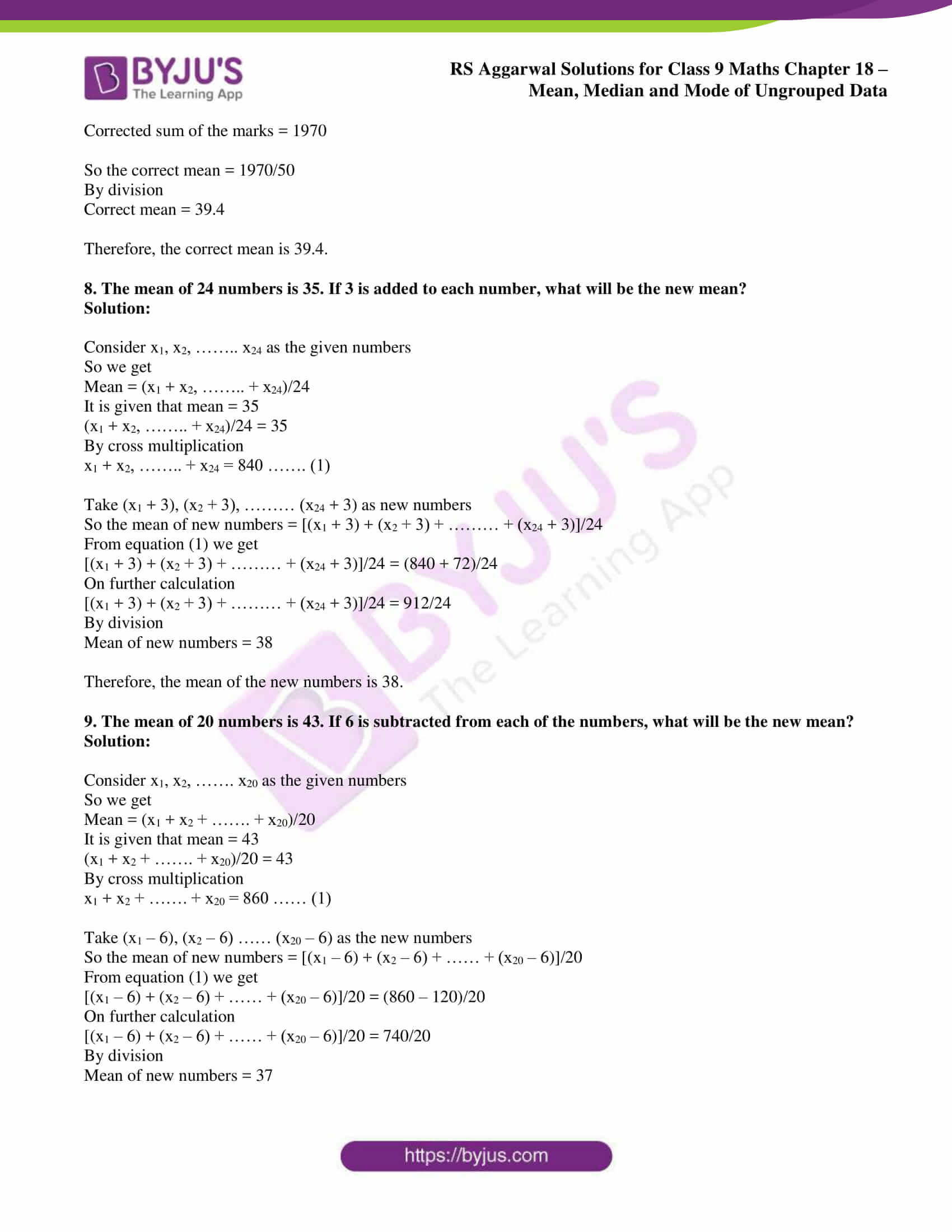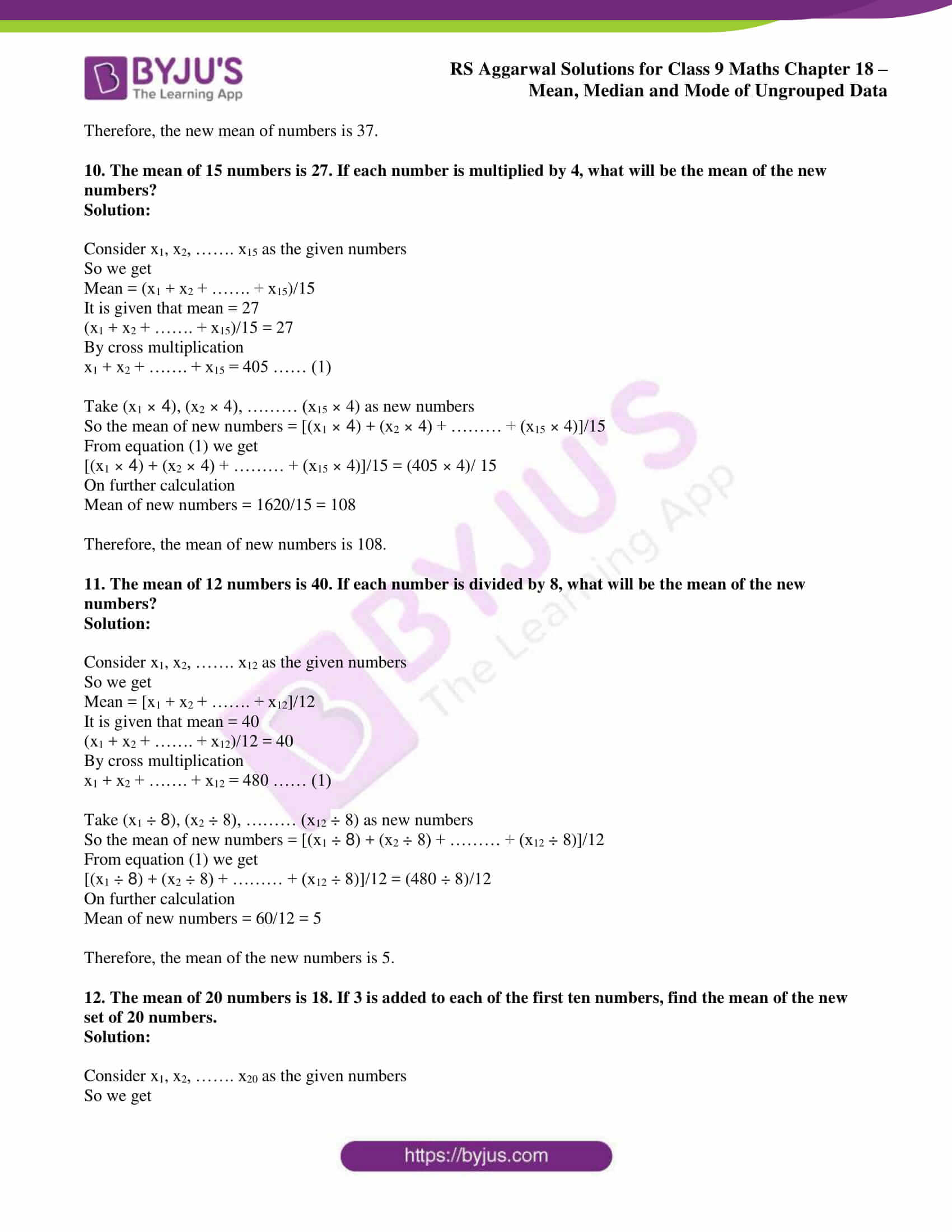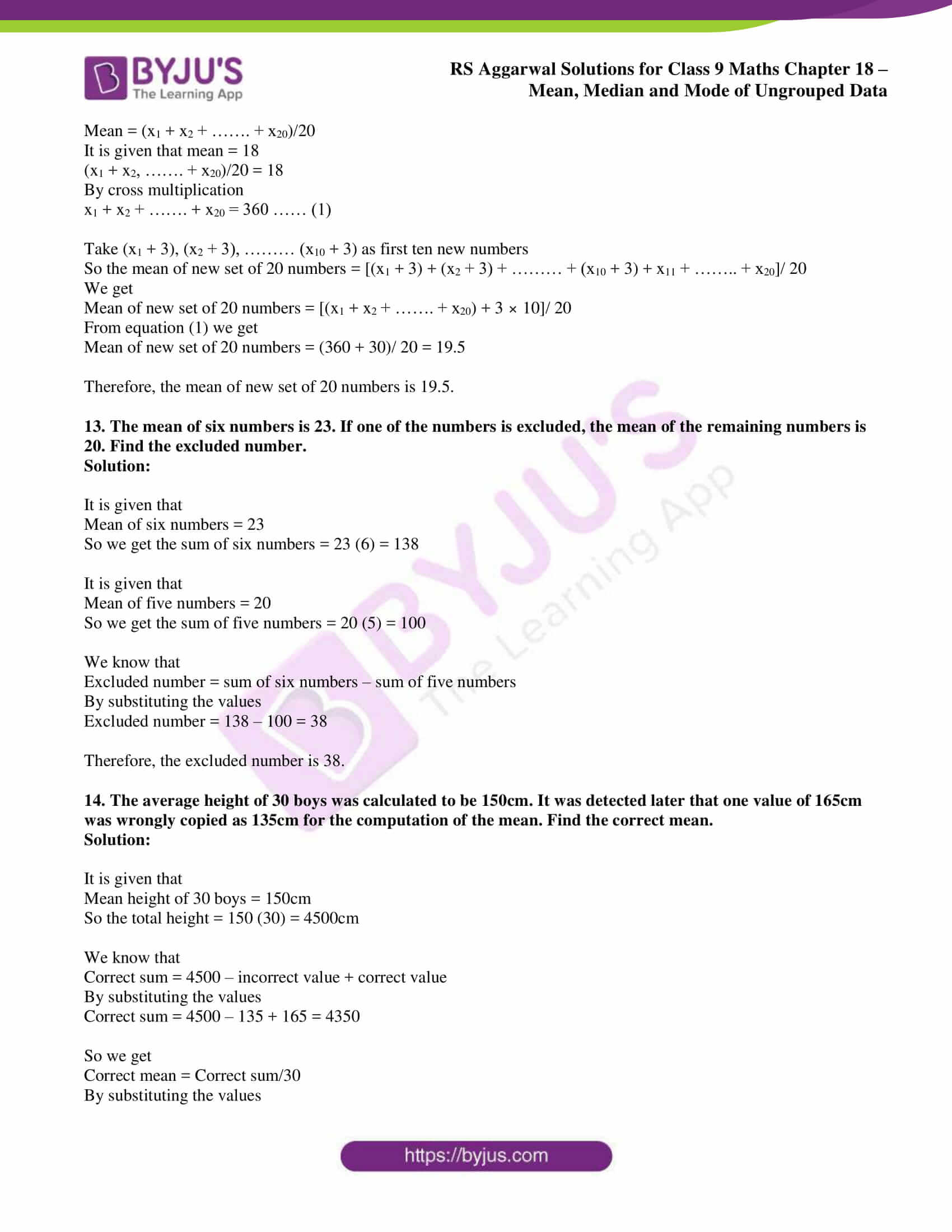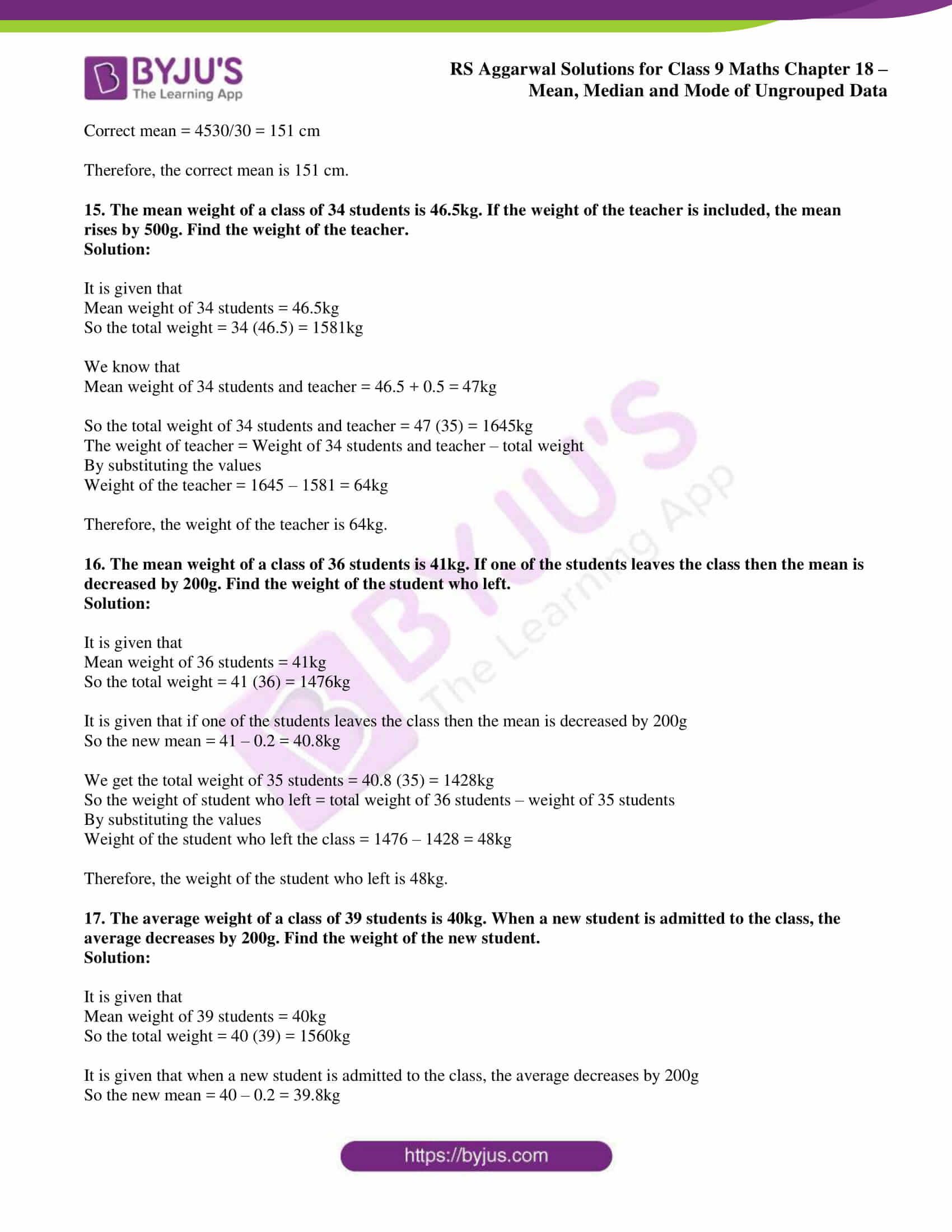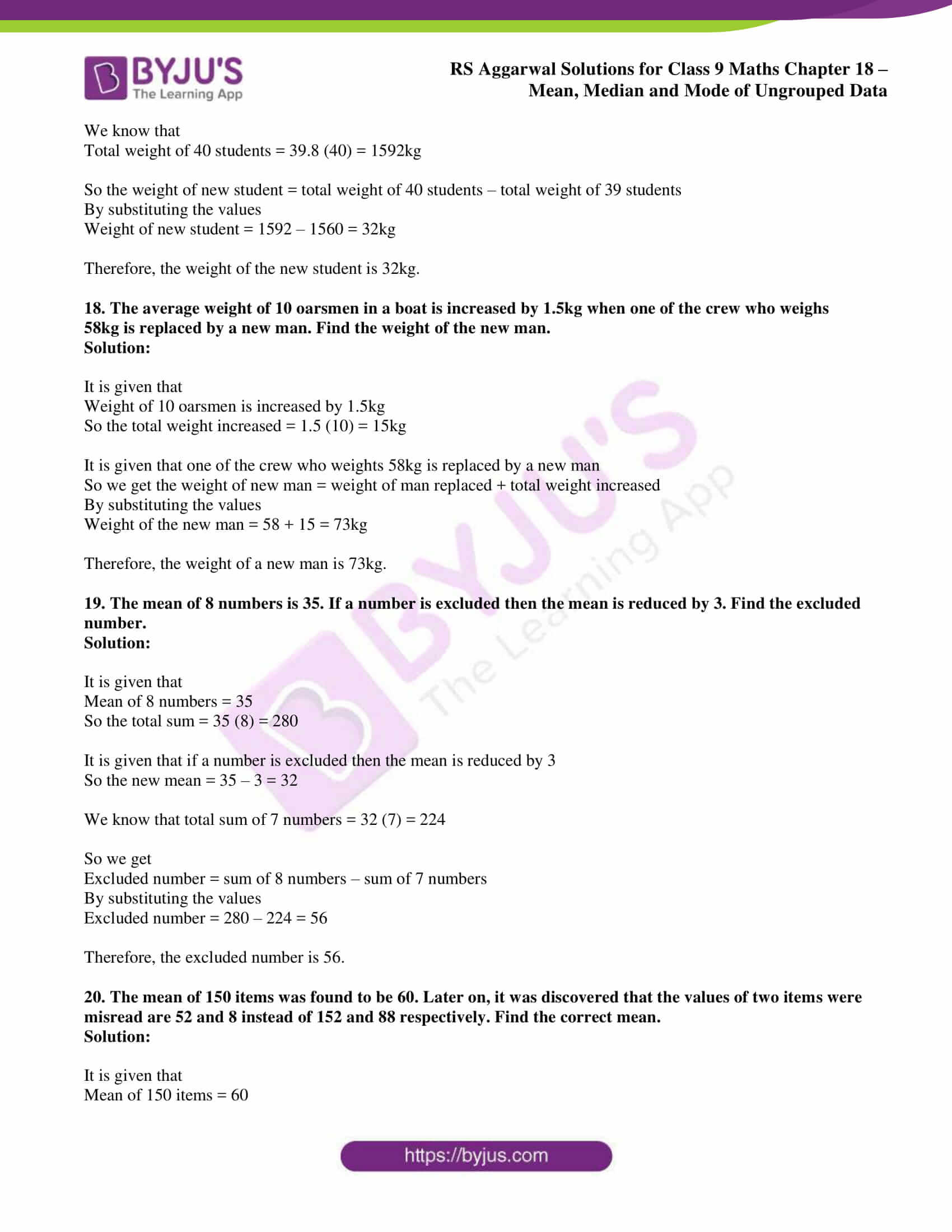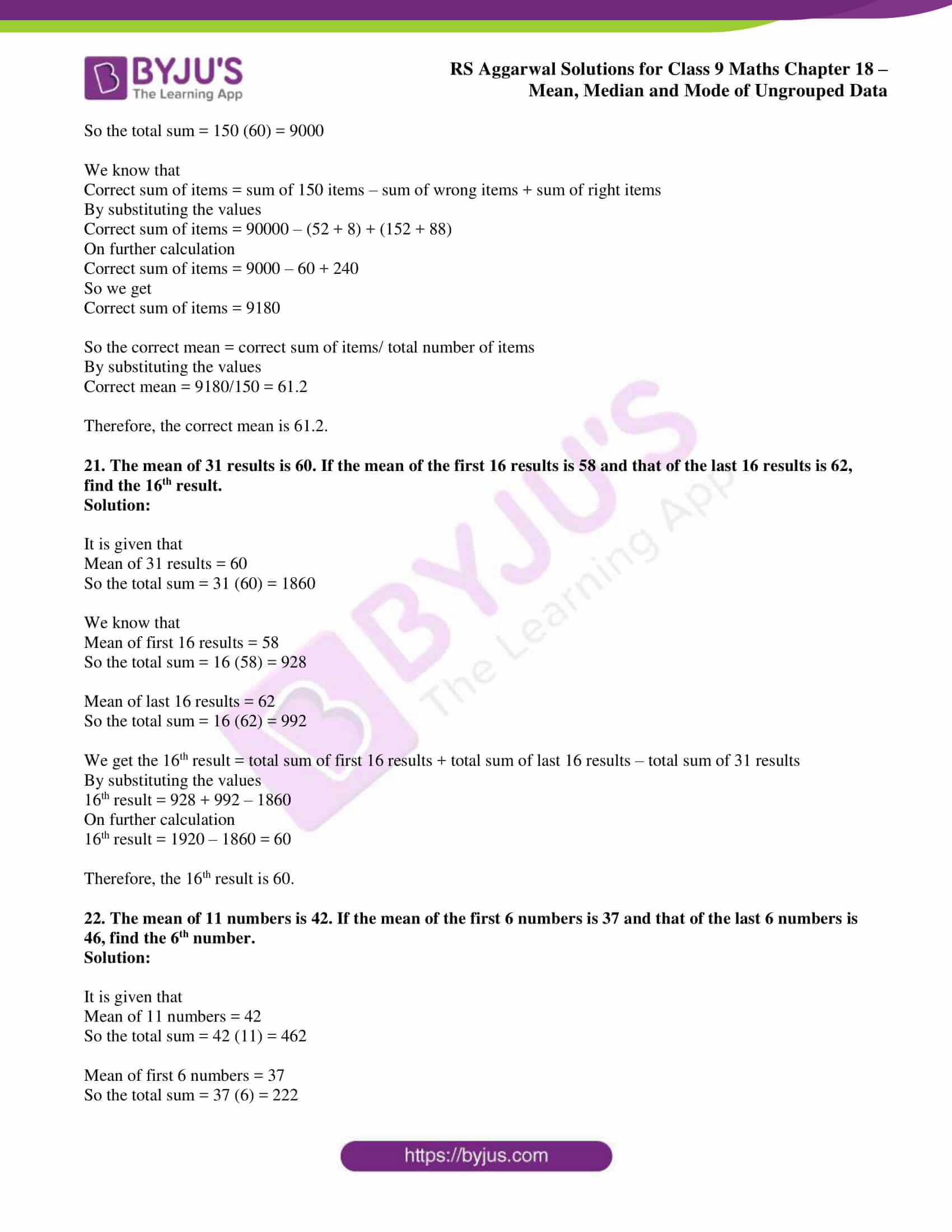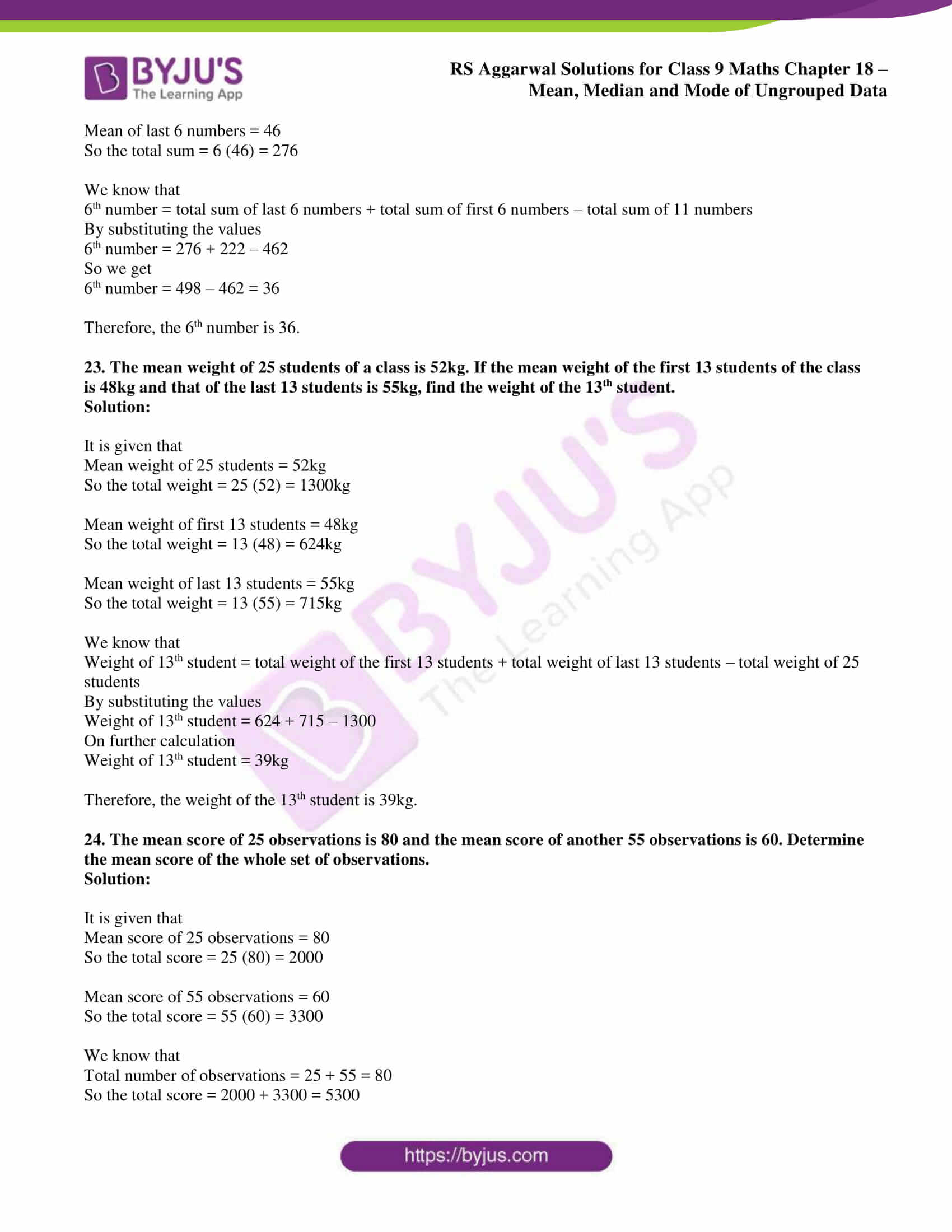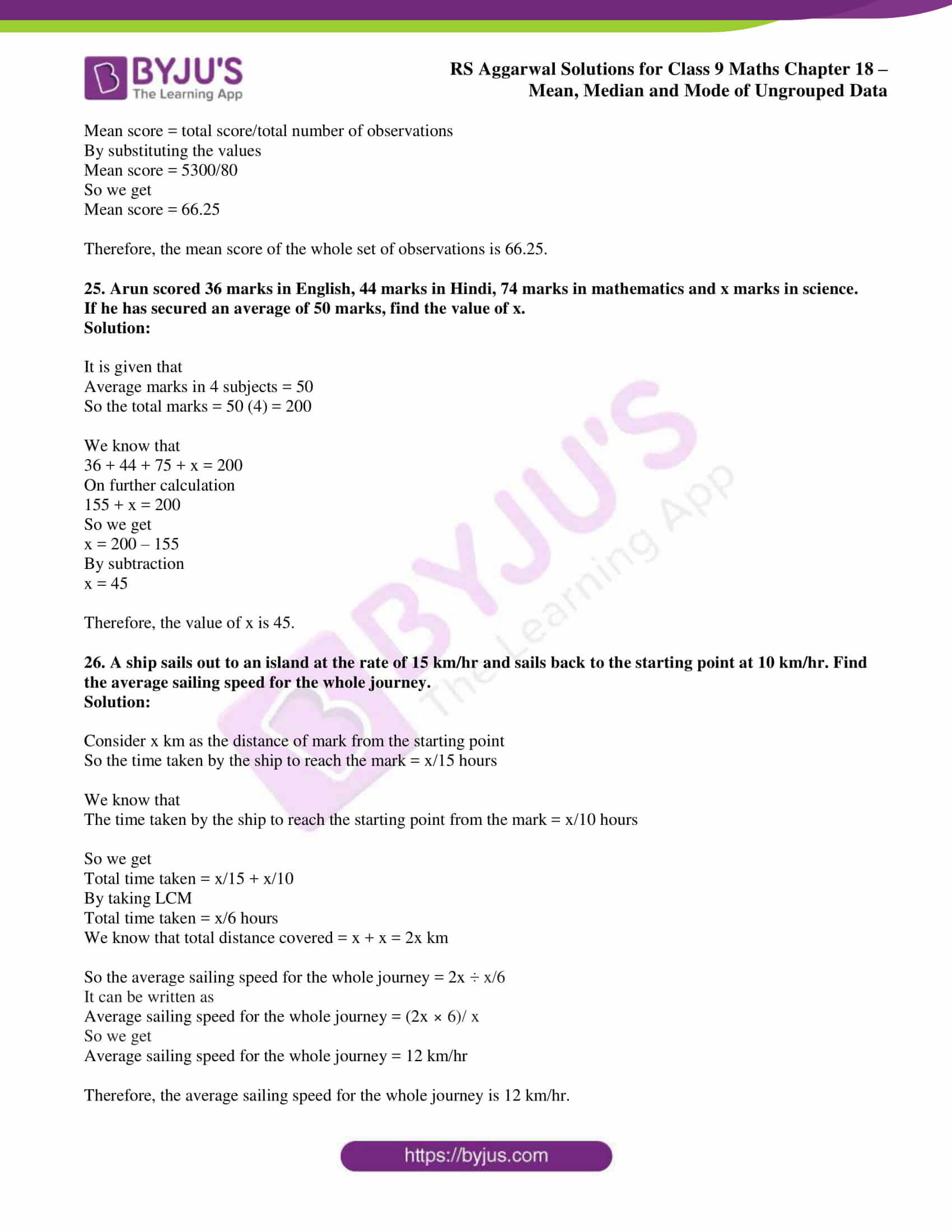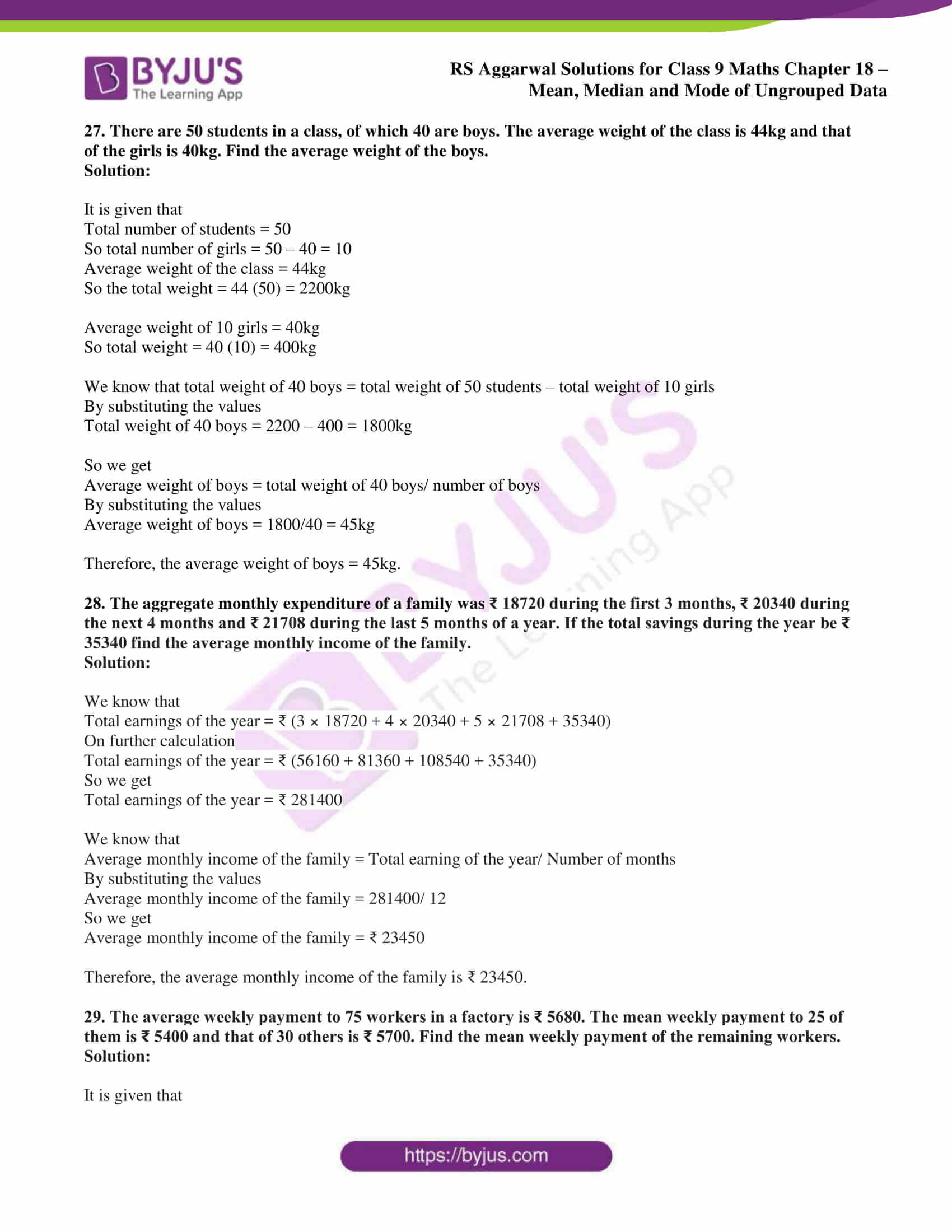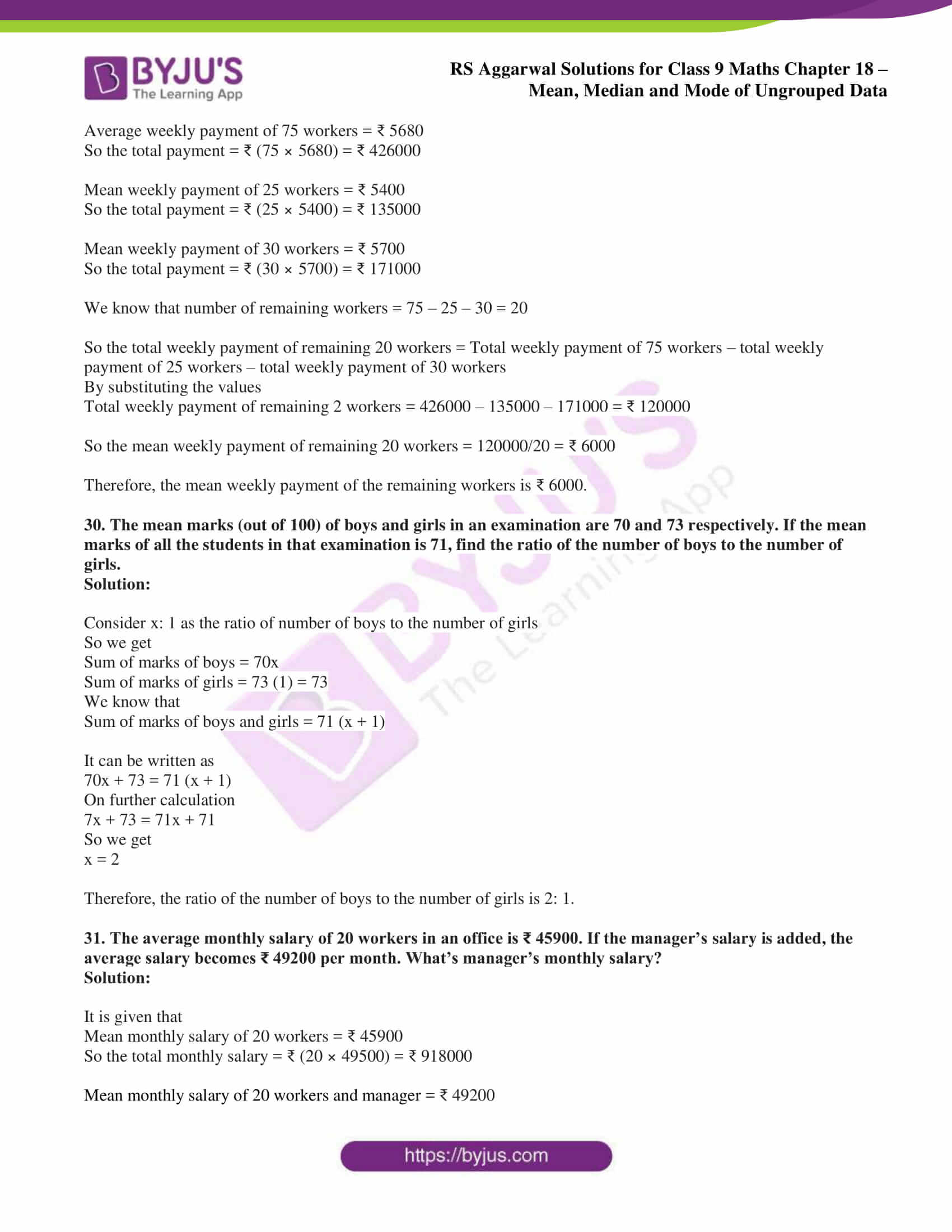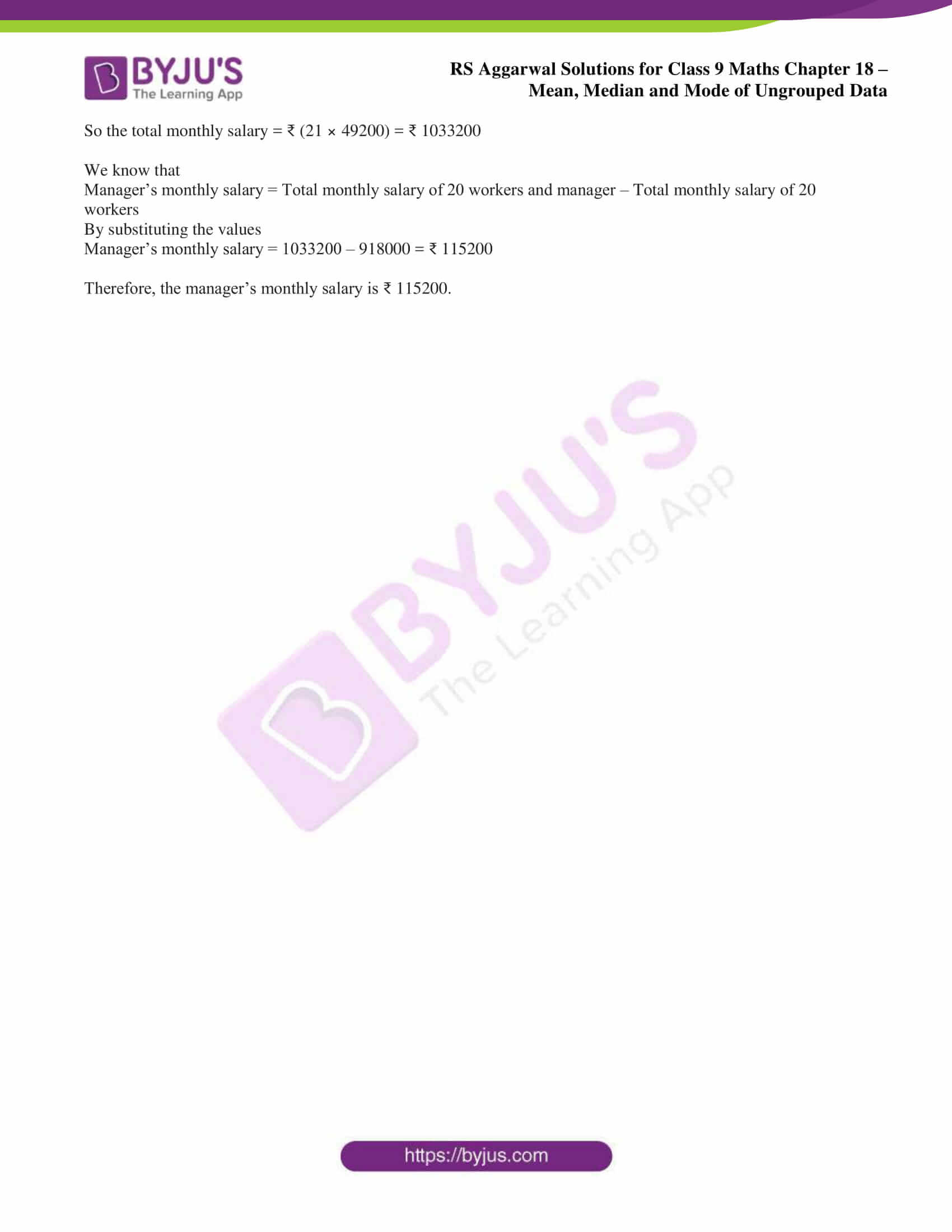## Access RS Aggarwal Solutions for Class 9 Chapter 18: Mean, Median and Mode of Ungrouped Data Exercise 18A

1. Find the mean of:

(i) the first eight natural numbers

(ii) the first ten odd numbers

(iii) the first seven multiples of 5

(iv) all the factors of 20

(v) all prime numbers between 50 and 80.

Solution:

(i) We know that

First eight natural numbers = 1, 2, 3, 4, 5, 6, 7 and 8

So we get

Mean = sum of numbers/ total numbers

By substituting the values

Mean = (1 + 2 + 3 + 4 + 5 + 6 + 7 + 8)/8

On further calculation

Mean = 36/8

By division

Mean = 4.5

Therefore, the mean of the first eight natural numbers is 4.5.

(ii) We know that

First ten odd numbers = 1, 3, 5, 7, 9, 11, 13, 15, 17 and 19

So we get

Mean = sum of numbers/ total numbers

By substituting the values

Mean = (1 + 3 + 5 + 7 + 9 + 11 + 13 + 15 + 17 + 19)/10

On further calculation

Mean = 100/10

By division

Mean = 10

Therefore, the mean of first ten odd numbers is 10.

(iii) We know that

First seven multiples of five = 5, 10, 15, 20, 25, 30 and 35

So we get

Mean = sum of numbers/ total numbers

By substituting the values

Mean = (5 + 10 + 15 + 20 + 25 + 30 + 35)/7

On further calculation

Mean = 140/7

By division

Mean = 20

Therefore, the mean of first seven multiples of five is 20.

(iv) We know that

All the factors of 20 = 1, 2, 4, 5, 10 and 20

So we get

Mean = sum of numbers/ total numbers

By substituting the values

Mean = (1 + 2 + 4 + 5 + 10 + 20)/6

On further calculation

Mean = 42/6

By division

Mean = 7

Therefore, the mean of all the factors of 20 is 7.

(v) We know that

All prime numbers between 50 and 80 = 53, 59, 61, 67, 71, 73 and 79

So we get

Mean = sum of numbers/ total numbers

By substituting the values

Mean = (53 + 59 + 61 + 67 + 71 + 73 + 79)/7

On further calculation

Mean = 463/7

So we get

Mean = 66 1/7

Therefore, the mean of all prime numbers between 50 and 80 is 66 1/7.

2. The number of children in 10 families of a locality are 2, 4, 3, 4, 2, 0, 3, 5, 1 and 6.

Find the mean number of children per family.

Solution:

It is given that number of children in 10 families of a locality are 2, 4, 3, 4, 2, 0, 3, 5, 1 and 6.

We know that

Mean = sum of numbers/ total numbers

By substituting the values

Mean = (2 + 4 + 3 + 4 + 2 + 0 + 3 + 5 + 1 + 6)/ 10

On further calculation

Mean = 30/10

By division

Mean = 3

Therefore, the mean number of children per family is 3.

3. The following are the numbers of books issued in a school library during a week:

105, 216, 322, 167, 273, 405 and 346.

Find the average number of books issued per day.

Solution:

It is given that the number of books issued are 105, 216, 322, 167, 273, 405 and 346.

We know that

Mean = sum of numbers/ total numbers

By substituting the values

Mean = (105 + 216 + 322 + 167 + 273 + 405 + 346)/ 7

On further calculation

Mean = 1834/7

By division

Mean = 262

Therefore, the average number of books issued per day is 262.

4. The daily minimum temperature recorded (in oF) at a place during six days of a week was as under:

 Monday 35.5 Tuesday 30.8 Wednesday 27.3 Thursday 32.1 Friday 23.8 Saturday 29.9

Find the mean temperature.

Solution:

We know that

Mean temperature = Sum of temperatures/ Number of days

By substituting the values

Mean temperature = (35.5 + 30.8 + 27.3 + 32.1 + 23.8 + 29.9)/6

On further calculation

Mean = 179.4/6

By division

Mean = 29.9 oF

Therefore, the mean temperature is 29.9 oF.

5. If the mean of five observations x, x + 2, x + 4, x + 6, x + 8 is 13, find the value of x and hence find the mean of the last three observations.

Solution:

We know that

Number of observations = 5

It is given that mean = 13

We can write it as

[x + (x + 2) + (x + 4) + (x + 6) + (x + 8)]/5 = 13

On further calculation

5x + 20 = 13 (5)

So we get

5x + 20 = 65

By subtraction

5x = 45

By division

x = 9

By substituting the value of x

We know that the last three observations are

9 + 4 = 13

9 + 6 = 15

9 + 8 = 17

We know that

Mean of last three observations = (13 + 15 + 17)/3

On further calculation

Mean of last three observations = 45/3

By division

Mean of last three observations = 15

Therefore, the mean of last three observations is 15.

6. The mean weight of 6 boys in a group is 48kg. The individual weights of five of them are 51kg, 45kg, 49kg, 46kg and 44kg. Find the weight of the sixth boy.

Solution:

It is given that

Mean weight of 6 boys = 48kg

So we get

Mean weight = sum of the weight of 6 boys/6 = 48

We know that

Sum of weight of six boys = 48 (6) = 288kg

So the sum of weight of 5 boys = (51 + 45 + 49 + 46 + 44) = 235kg

We get

Weight of sixth boy = sum of weight of six boys – sum of weight of 5 boys

By substituting the values

Weight of sixth boy = 288 – 235

By subtraction

Weight of sixth boy = 53kg

Therefore, the weight of sixth boy is 53 kg.

7. The mean of the marks scored by 50 students was found to be 39. Later on it was discovered that a score of 43 was misread as 23. Find the correct mean.

Solution:

It is given that

Mean of the marks of 50 students = 39

So we get

Sum of these marks = 39 (50) = 1950

We can write it as

Corrected sum of the marks = 1950 – wrong number + correct number

By substituting the values

Corrected sum of the marks = 1950 – 23 + 43

So we get

Corrected sum of the marks = 1970

So the correct mean = 1970/50

By division

Correct mean = 39.4

Therefore, the correct mean is 39.4.

8. The mean of 24 numbers is 35. If 3 is added to each number, what will be the new mean?

Solution:

Consider x1, x2, …….. x24 as the given numbers

So we get

Mean = (x1 + x2, …….. + x24)/24

It is given that mean = 35

(x1 + x2, …….. + x24)/24 = 35

By cross multiplication

x1 + x2, …….. + x24 = 840 ……. (1)

Take (x1 + 3), (x2 + 3), ……… (x24 + 3) as new numbers

So the mean of new numbers = [(x1 + 3) + (x2 + 3) + ……… + (x24 + 3)]/24

From equation (1) we get

[(x1 + 3) + (x2 + 3) + ……… + (x24 + 3)]/24 = (840 + 72)/24

On further calculation

[(x1 + 3) + (x2 + 3) + ……… + (x24 + 3)]/24 = 912/24

By division

Mean of new numbers = 38

Therefore, the mean of the new numbers is 38.

9. The mean of 20 numbers is 43. If 6 is subtracted from each of the numbers, what will be the new mean?

Solution:

Consider x1, x2, ……. x20 as the given numbers

So we get

Mean = (x1 + x2 + ……. + x20)/20

It is given that mean = 43

(x1 + x2 + ……. + x20)/20 = 43

By cross multiplication

x1 + x2 + ……. + x20 = 860 …… (1)

Take (x1 – 6), (x2 – 6) …… (x20 – 6) as the new numbers

So the mean of new numbers = [(x1 – 6) + (x2 – 6) + …… + (x20 – 6)]/20

From equation (1) we get

[(x1 – 6) + (x2 – 6) + …… + (x20 – 6)]/20 = (860 – 120)/20

On further calculation

[(x1 – 6) + (x2 – 6) + …… + (x20 – 6)]/20 = 740/20

By division

Mean of new numbers = 37

Therefore, the new mean of numbers is 37.

10. The mean of 15 numbers is 27. If each number is multiplied by 4, what will be the mean of the new numbers?

Solution:

Consider x1, x2, ……. x15 as the given numbers

So we get

Mean = (x1 + x2 + ……. + x15)/15

It is given that mean = 27

(x1 + x2 + ……. + x15)/15 = 27

By cross multiplication

x1 + x2 + ……. + x15 = 405 …… (1)

Take (x1 × 4), (x2 × 4), ……… (x15 × 4) as new numbers

So the mean of new numbers = [(x1 × 4) + (x2 × 4) + ……… + (x15 × 4)]/15

From equation (1) we get

[(x1 × 4) + (x2 × 4) + ……… + (x15 × 4)]/15 = (405 × 4)/ 15

On further calculation

Mean of new numbers = 1620/15 = 108

Therefore, the mean of new numbers is 108.

11. The mean of 12 numbers is 40. If each number is divided by 8, what will be the mean of the new numbers?

Solution:

Consider x1, x2, ……. x12 as the given numbers

So we get

Mean = [x1 + x2 + ……. + x12]/12

It is given that mean = 40

(x1 + x2 + ……. + x12)/12 = 40

By cross multiplication

x1 + x2 + ……. + x12 = 480 …… (1)

Take (x1 ÷ 8), (x2 ÷ 8), ……… (x12 ÷ 8) as new numbers

So the mean of new numbers = [(x1 ÷ 8) + (x2 ÷ 8) + ……… + (x12 ÷ 8)]/12

From equation (1) we get

[(x1 ÷ 8) + (x2 ÷ 8) + ……… + (x12 ÷ 8)]/12 = (480 ÷ 8)/12

On further calculation

Mean of new numbers = 60/12 = 5

Therefore, the mean of the new numbers is 5.

12. The mean of 20 numbers is 18. If 3 is added to each of the first ten numbers, find the mean of the new set of 20 numbers.

Solution:

Consider x1, x2, ……. x20 as the given numbers

So we get

Mean = (x1 + x2 + ……. + x20)/20

It is given that mean = 18

(x1 + x2, ……. + x20)/20 = 18

By cross multiplication

x1 + x2 + ……. + x20 = 360 …… (1)

Take (x1 + 3), (x2 + 3), ……… (x10 + 3) as first ten new numbers

So the mean of new set of 20 numbers = [(x1 + 3) + (x2 + 3) + ……… + (x10 + 3) + x11 + …….. + x20]/ 20

We get

Mean of new set of 20 numbers = [(x1 + x2 + ……. + x20) + 3 × 10]/ 20

From equation (1) we get

Mean of new set of 20 numbers = (360 + 30)/ 20 = 19.5

Therefore, the mean of new set of 20 numbers is 19.5.

13. The mean of six numbers is 23. If one of the numbers is excluded, the mean of the remaining numbers is 20. Find the excluded number.

Solution:

It is given that

Mean of six numbers = 23

So we get the sum of six numbers = 23 (6) = 138

It is given that

Mean of five numbers = 20

So we get the sum of five numbers = 20 (5) = 100

We know that

Excluded number = sum of six numbers – sum of five numbers

By substituting the values

Excluded number = 138 – 100 = 38

Therefore, the excluded number is 38.

14. The average height of 30 boys was calculated to be 150cm. It was detected later that one value of 165cm was wrongly copied as 135cm for the computation of the mean. Find the correct mean.

Solution:

It is given that

Mean height of 30 boys = 150cm

So the total height = 150 (30) = 4500cm

We know that

Correct sum = 4500 – incorrect value + correct value

By substituting the values

Correct sum = 4500 – 135 + 165 = 4350

So we get

Correct mean = Correct sum/30

By substituting the values

Correct mean = 4530/30 = 151 cm

Therefore, the correct mean is 151 cm.

15. The mean weight of a class of 34 students is 46.5kg. If the weight of the teacher is included, the mean rises by 500g. Find the weight of the teacher.

Solution:

It is given that

Mean weight of 34 students = 46.5kg

So the total weight = 34 (46.5) = 1581kg

We know that

Mean weight of 34 students and teacher = 46.5 + 0.5 = 47kg

So the total weight of 34 students and teacher = 47 (35) = 1645kg

The weight of teacher = Weight of 34 students and teacher – total weight

By substituting the values

Weight of the teacher = 1645 – 1581 = 64kg

Therefore, the weight of the teacher is 64kg.

16. The mean weight of a class of 36 students is 41kg. If one of the students leaves the class then the mean is decreased by 200g. Find the weight of the student who left.

Solution:

It is given that

Mean weight of 36 students = 41kg

So the total weight = 41 (36) = 1476kg

It is given that if one of the students leaves the class then the mean is decreased by 200g

So the new mean = 41 – 0.2 = 40.8kg

We get the total weight of 35 students = 40.8 (35) = 1428kg

So the weight of student who left = total weight of 36 students – weight of 35 students

By substituting the values

Weight of the student who left the class = 1476 – 1428 = 48kg

Therefore, the weight of the student who left is 48kg.

17. The average weight of a class of 39 students is 40kg. When a new student is admitted to the class, the average decreases by 200g. Find the weight of the new student.

Solution:

It is given that

Mean weight of 39 students = 40kg

So the total weight = 40 (39) = 1560kg

It is given that when a new student is admitted to the class, the average decreases by 200g

So the new mean = 40 – 0.2 = 39.8kg

We know that

Total weight of 40 students = 39.8 (40) = 1592kg

So the weight of new student = total weight of 40 students – total weight of 39 students

By substituting the values

Weight of new student = 1592 – 1560 = 32kg

Therefore, the weight of the new student is 32kg.

18. The average weight of 10 oarsmen in a boat is increased by 1.5kg when one of the crew who weighs 58kg is replaced by a new man. Find the weight of the new man.

Solution:

It is given that

Weight of 10 oarsmen is increased by 1.5kg

So the total weight increased = 1.5 (10) = 15kg

It is given that one of the crew who weights 58kg is replaced by a new man

So we get the weight of new man = weight of man replaced + total weight increased

By substituting the values

Weight of the new man = 58 + 15 = 73kg

Therefore, the weight of a new man is 73kg.

19. The mean of 8 numbers is 35. If a number is excluded then the mean is reduced by 3. Find the excluded number.

Solution:

It is given that

Mean of 8 numbers = 35

So the total sum = 35 (8) = 280

It is given that if a number is excluded then the mean is reduced by 3

So the new mean = 35 – 3 = 32

We know that total sum of 7 numbers = 32 (7) = 224

So we get

Excluded number = sum of 8 numbers – sum of 7 numbers

By substituting the values

Excluded number = 280 – 224 = 56

Therefore, the excluded number is 56.

20. The mean of 150 items was found to be 60. Later on, it was discovered that the values of two items were misread are 52 and 8 instead of 152 and 88 respectively. Find the correct mean.

Solution:

It is given that

Mean of 150 items = 60

So the total sum = 150 (60) = 9000

We know that

Correct sum of items = sum of 150 items – sum of wrong items + sum of right items

By substituting the values

Correct sum of items = 90000 – (52 + 8) + (152 + 88)

On further calculation

Correct sum of items = 9000 – 60 + 240

So we get

Correct sum of items = 9180

So the correct mean = correct sum of items/ total number of items

By substituting the values

Correct mean = 9180/150 = 61.2

Therefore, the correct mean is 61.2.

21. The mean of 31 results is 60. If the mean of the first 16 results is 58 and that of the last 16 results is 62, find the 16th result.

Solution:

It is given that

Mean of 31 results = 60

So the total sum = 31 (60) = 1860

We know that

Mean of first 16 results = 58

So the total sum = 16 (58) = 928

Mean of last 16 results = 62

So the total sum = 16 (62) = 992

We get the 16th result = total sum of first 16 results + total sum of last 16 results – total sum of 31 results

By substituting the values

16th result = 928 + 992 – 1860

On further calculation

16th result = 1920 – 1860 = 60

Therefore, the 16th result is 60.

22. The mean of 11 numbers is 42. If the mean of the first 6 numbers is 37 and that of the last 6 numbers is 46, find the 6th number.

Solution:

It is given that

Mean of 11 numbers = 42

So the total sum = 42 (11) = 462

Mean of first 6 numbers = 37

So the total sum = 37 (6) = 222

Mean of last 6 numbers = 46

So the total sum = 6 (46) = 276

We know that

6th number = total sum of last 6 numbers + total sum of first 6 numbers – total sum of 11 numbers

By substituting the values

6th number = 276 + 222 – 462

So we get

6th number = 498 – 462 = 36

Therefore, the 6th number is 36.

23. The mean weight of 25 students of a class is 52kg. If the mean weight of the first 13 students of the class is 48kg and that of the last 13 students is 55kg, find the weight of the 13th student.

Solution:

It is given that

Mean weight of 25 students = 52kg

So the total weight = 25 (52) = 1300kg

Mean weight of first 13 students = 48kg

So the total weight = 13 (48) = 624kg

Mean weight of last 13 students = 55kg

So the total weight = 13 (55) = 715kg

We know that

Weight of 13th student = total weight of the first 13 students + total weight of last 13 students – total weight of 25 students

By substituting the values

Weight of 13th student = 624 + 715 – 1300

On further calculation

Weight of 13th student = 39kg

Therefore, the weight of the 13th student is 39kg.

24. The mean score of 25 observations is 80 and the mean score of another 55 observations is 60. Determine the mean score of the whole set of observations.

Solution:

It is given that

Mean score of 25 observations = 80

So the total score = 25 (80) = 2000

Mean score of 55 observations = 60

So the total score = 55 (60) = 3300

We know that

Total number of observations = 25 + 55 = 80

So the total score = 2000 + 3300 = 5300

Mean score = total score/total number of observations

By substituting the values

Mean score = 5300/80

So we get

Mean score = 66.25

Therefore, the mean score of the whole set of observations is 66.25.

25. Arun scored 36 marks in English, 44 marks in Hindi, 74 marks in mathematics and x marks in science. If he has secured an average of 50 marks, find the value of x.

Solution:

It is given that

Average marks in 4 subjects = 50

So the total marks = 50 (4) = 200

We know that

36 + 44 + 75 + x = 200

On further calculation

155 + x = 200

So we get

x = 200 – 155

By subtraction

x = 45

Therefore, the value of x is 45.

26. A ship sails out to an island at the rate of 15 km/hr and sails back to the starting point at 10 km/hr. Find the average sailing speed for the whole journey.

Solution:

Consider x km as the distance of mark from the starting point

So the time taken by the ship to reach the mark = x/15 hours

We know that

The time taken by the ship to reach the starting point from the mark = x/10 hours

So we get

Total time taken = x/15 + x/10

By taking LCM

Total time taken = x/6 hours

We know that total distance covered = x + x = 2x km

So the average sailing speed for the whole journey = 2x ÷ x/6

It can be written as

Average sailing speed for the whole journey = (2x × 6)/ x

So we get

Average sailing speed for the whole journey = 12 km/hr

Therefore, the average sailing speed for the whole journey is 12 km/hr.

27. There are 50 students in a class, of which 40 are boys. The average weight of the class is 44kg and that of the girls is 40kg. Find the average weight of the boys.

Solution:

It is given that

Total number of students = 50

So total number of girls = 50 – 40 = 10

Average weight of the class = 44kg

So the total weight = 44 (50) = 2200kg

Average weight of 10 girls = 40kg

So total weight = 40 (10) = 400kg

We know that total weight of 40 boys = total weight of 50 students – total weight of 10 girls

By substituting the values

Total weight of 40 boys = 2200 – 400 = 1800kg

So we get

Average weight of boys = total weight of 40 boys/ number of boys

By substituting the values

Average weight of boys = 1800/40 = 45kg

Therefore, the average weight of boys = 45kg.

28. The aggregate monthly expenditure of a family was ₹ 18720 during the first 3 months, ₹ 20340 during the next 4 months and ₹ 21708 during the last 5 months of a year. If the total savings during the year be ₹ 35340 find the average monthly income of the family.

Solution:

We know that

Total earnings of the year = ₹ (3 × 18720 + 4 × 20340 + 5 × 21708 + 35340)

On further calculation

Total earnings of the year = ₹ (56160 + 81360 + 108540 + 35340)

So we get

Total earnings of the year = ₹ 281400

We know that

Average monthly income of the family = Total earning of the year/ Number of months

By substituting the values

Average monthly income of the family = 281400/ 12

So we get

Average monthly income of the family = ₹ 23450

Therefore, the average monthly income of the family is ₹ 23450.

29. The average weekly payment to 75 workers in a factory is ₹ 5680. The mean weekly payment to 25 of them is ₹ 5400 and that of 30 others is ₹ 5700. Find the mean weekly payment of the remaining workers.

Solution:

It is given that

Average weekly payment of 75 workers = ₹ 5680

So the total payment = ₹ (75 × 5680) = ₹ 426000

Mean weekly payment of 25 workers = ₹ 5400

So the total payment = ₹ (25 × 5400) = ₹ 135000

Mean weekly payment of 30 workers = ₹ 5700

So the total payment = ₹ (30 × 5700) = ₹ 171000

We know that number of remaining workers = 75 – 25 – 30 = 20

So the total weekly payment of remaining 20 workers = Total weekly payment of 75 workers – total weekly payment of 25 workers – total weekly payment of 30 workers

By substituting the values

Total weekly payment of remaining 2 workers = 426000 – 135000 – 171000 = ₹ 120000

So the mean weekly payment of remaining 20 workers = 120000/20 = ₹ 6000

Therefore, the mean weekly payment of the remaining workers is ₹ 6000.

30. The mean marks (out of 100) of boys and girls in an examination are 70 and 73 respectively. If the mean marks of all the students in that examination is 71, find the ratio of the number of boys to the number of girls.

Solution:

Consider x: 1 as the ratio of number of boys to the number of girls

So we get

Sum of marks of boys = 70x

Sum of marks of girls = 73 (1) = 73

We know that

Sum of marks of boys and girls = 71 (x + 1)

It can be written as

70x + 73 = 71 (x + 1)

On further calculation

7x + 73 = 71x + 71

So we get

x = 2

Therefore, the ratio of the number of boys to the number of girls is 2: 1.

31. The average monthly salary of 20 workers in an office is ₹ 45900. If the manager’s salary is added, the average salary becomes ₹ 49200 per month. What’s manager’s monthly salary?

Solution:

It is given that

Mean monthly salary of 20 workers = ₹ 45900

So the total monthly salary = ₹ (20 × 49500) = ₹ 918000

Mean monthly salary of 20 workers and manager = ₹ 49200

So the total monthly salary = ₹ (21 × 49200) = ₹ 1033200

We know that

Manager’s monthly salary = Total monthly salary of 20 workers and manager – Total monthly salary of 20 workers

By substituting the values

Manager’s monthly salary = 1033200 – 918000 = ₹ 115200

Therefore, the manager’s monthly salary is ₹ 115200.

### Access other exercise solutions of Class 9 Maths Chapter 18: Mean, Median and Mode of Ungrouped Data

Exercise 18B Solutions 14 Questions

Exercise 18C Solutions 10 Questions

Exercise 18D Solutions 8 Questions

### RS Aggarwal Solutions Class 9 Maths Chapter 18 – Mean, Median and Mode of Ungrouped Data Exercise 18A

RS Aggarwal Solutions Class 9 Maths Chapter 18 Mean, Median and Mode of Ungrouped Data Exercise 18A consists of various methods and formulas which can be used in finding mode of given data.

### Key features of RS Aggarwal Solutions for Class 9 Maths Chapter 18: Mean, Median and Mode of Ungrouped Data Exercise 18A

• The solutions are provided by experienced faculty after vast research conducted on each topic.
• The exercise wise problems can be solved using the PDF as a guide to score better marks in the board exam.
• The answers are accurate in a stepwise manner based on the latest CBSE guidelines and weightage for each concept.
• The main aim of preparing solutions is to improve confidence among students to appear for board exams.